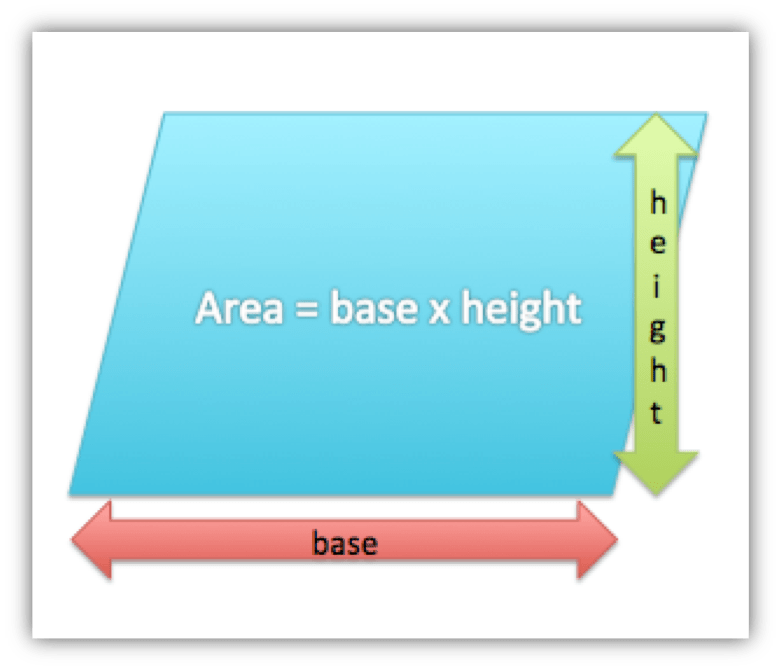# Area of Rectangles and Parallelograms

In life, we measure many things for different reasons. For example, a carpenter is going to install a new floor in a kitchen. The carpenter needs to measure the length and width of the kitchen to find the area. The carpenter wants to order the right amount of flooring. Thus, the carpenter wants to be exact and use the correct units when measuring.

Before we talk about what units are appropriate, we need to define what we could be measuring.## Area

The area of a figure is the number of square units it takes to completely fill a space or surface.   Area is measured in square units.

## Area of a Rectangle

To find the area of a rectangle, multiply the length by the width.## Area of a Parallelogram

To find the area of a parallelogram, multiply the base by the height.## Perimeter

The perimeter of a shape is the measure around it, the lengths of all the sides added together.

(source)